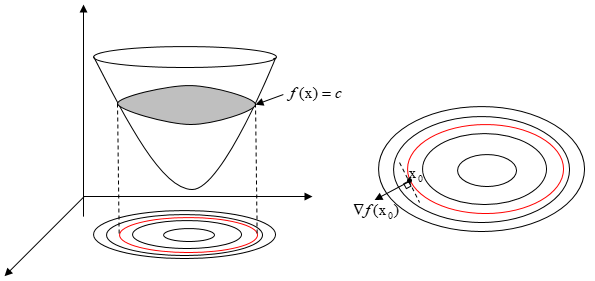# 深度学习中的优化方法（二）

#### 一、常用的优化算法总结

2. 二阶方法：牛顿法、拟牛顿法、共轭梯度法（CG）、BFGS、L-BFGS。

#### 2.1 梯度下降法的基本原理$\text{x}^{k+1} = \text{x}^{k}-\alpha_{k}\nabla f(\text{x}^{k})$

#### 2.2 梯度下降法的具体实现

$J(\Theta) = \dfrac{1}{2m}\sum_{i=1}^{m}[f_{\Theta}(x^{i})-y^{i}]^{2}$

$\dfrac{\partial J(\Theta)}{\partial \Theta_{j}} = \dfrac{1}{m}\sum_{i=1}^{m}[f_{\Theta}(x^{i})-y^{i}] \times \dfrac{\partial f_{\Theta}(x^{i})}{\partial \Theta_{j}} = \dfrac{1}{m}\nabla f_{\Theta}(x^{i})\sum_{i=1}^{m}[f_{\Theta}(x^{i})-y^{i}]$

$\Theta_{j} := \Theta_{j}-\alpha \dfrac{1}{m}\nabla f_{\Theta}(x^{i})\sum_{i=1}^{m}[f_{\Theta}(x^{i})-y^{i}]$

#### 2.2.1 随机梯度下降（SGD）

$\Theta_{j} := \Theta_{j}-\alpha\nabla f_{\Theta}(x^{i})[f_{\Theta}(x^{i})-y^{i}]$

for i in range(nb_epochs):
np.random.shuffle(data)
for example in data:
params = params - learning_rate * params_grad


#### 2.2.2 批量梯度下降 （BGD）

for i in range(nb_epochs):
params = params - learning_rate * params_grad


#### 2.2.3 部分批量梯度下降（mini-batch GD）

for i in range(nb_epochs):
np.random.shuffle(data)
for batch in get_batches(data, batch_size=50):
params = params - learning_rate * params_grad


#### 2.3.1 Momentum

\begin{aligned} v_{t} &=\gamma v_{t-1}+\eta \nabla_{\theta} J(\theta) \\ \theta &=\theta-v_{t} \end{aligned}

$\theta_{t+1, i}=\theta_{t, i}-\frac{\eta}{\sqrt{G_{t, i i}+\epsilon}} \cdot g_{t, i}$

$g_{t, i}=\nabla_{\theta} J\left(\theta_{i}\right)$

$\theta_{t+1, i}=\theta_{t, i}-\eta \cdot g_{t, i}$

$\theta_{t+1, i}=\theta_{t, i}-\frac{\eta}{\sqrt{G_{t, i i}+\epsilon}} \cdot g_{t, i}$

#### 2.3.3 RMSProp

$\begin{array}{l} \theta_{t+1}=\theta_{t}-\frac{\eta}{\sqrt{E\left[g^{2}\right]_{t}+\epsilon}} g_{t} \end{array}$

$E\left[g^{2}\right]_{t}=0.9 E\left[g^{2}\right]_{t-1}+0.1 g_{t}^{2}$

$\begin{array}{l} m_{t}=\beta_{1} m_{t-1}+\left(1-\beta_{1}\right) g_{t} \\ v_{t}=\beta_{2} v_{t-1}+\left(1-\beta_{2}\right) g_{t}^{2} \end{array}$

$\begin{array}{l} \hat{m}_{t}=\frac{m_{t}}{1-\beta_{1}^{t}} \\ \hat{v}_{t}=\frac{v_{t}}{1-\beta_{2}^{t}} \end{array}$

$\theta_{t+1}=\theta_{t}-\frac{\eta}{\sqrt{\hat{v}_{t}}+\epsilon} \hat{m}_{t}$

#### 三、牛顿法

$f(x) \approx f(x^{k}) + (x-x^{k})^{T}\nabla f(x^{k}) + \dfrac{1}{2}(x-x^{k})^{T}F(x^{k})(x-x^{k})$

$\dfrac{\partial f(x)}{\partial x} = \nabla f(x^{k}) + F(x^{k})(x-x^{k}) = 0$

$x^{k+1} = x^{k}-F^{-1}(x^{k})\nabla f(x^{k})$

$H=\begin{bmatrix} A&B\\ B&C\\ \end{bmatrix}=\begin{bmatrix} \dfrac{\partial^{2} f(x)}{\partial x^{2}}&\dfrac{\partial^{2} f(x)}{\partial xy}\\ \dfrac{\partial^{2} f(x)}{\partial xy}&\dfrac{\partial^{2} f(x)}{\partial y^{2}}\\ \end{bmatrix}$

1. $f'(x_{0}，y_{0})=0$
2. $|H|>0$$A>0$，为极小值；$|H|>0$$A<0$，为极大值；

1. $f'(x_{1}, x_{2},...x_{n})=0$
2. $|H|>0$$H$ 的顺序主子式均大于 0 ，为极小值（$H$ 为正定矩阵）；$|H|>0$ 且 ，且 $H$ 的顺序主子式均小于 0（$H$ 为负定矩阵），为极大值；

1. 对目标函数有较严格的要求。函数必须具有连续的一、二阶偏导数，海海森矩阵必须正定。
2. 计算相当复杂，除需要计算梯度以外，还需要计算二阶偏导数矩阵和它的逆矩阵。计算量、存储量均很⼤，且均以维数N的平⽅增加，当N很⼤时这个问题更加突出。

⽜顿法虽然收敛速度快，但是计算过程中需要计算目标函数的二阶偏导数，计算复杂度较⼤。而且有时目标函数的海森矩阵无法保持正定，从而使⽜顿法失效。为了克服这两个问题，⼈们提出了拟⽜牛顿法。这个方法的基本思想是：不⽤⼆阶偏导数而构造出可以近似海森矩阵或者海森矩阵的逆的正定对称阵，在拟⽜顿的条件下优化⽬目标函数。不同的构造⽅法就产生了不同的拟牛顿法。

#### 四、参考资料

《最优化导论第四版》孙志强，白圣建等译

CV总复习-深度学习机器学习基础篇（一），作者灯会

https://zhuanlan.zhihu.com/p/32230623

https://www.cnblogs.com/bigmonkey/p/8319082.html

https://blog.csdn.net/qq_34562355/article/details/109225384

posted @ 2021-08-04 13:31  ZhiboZhao  阅读(207)  评论(0编辑  收藏  举报